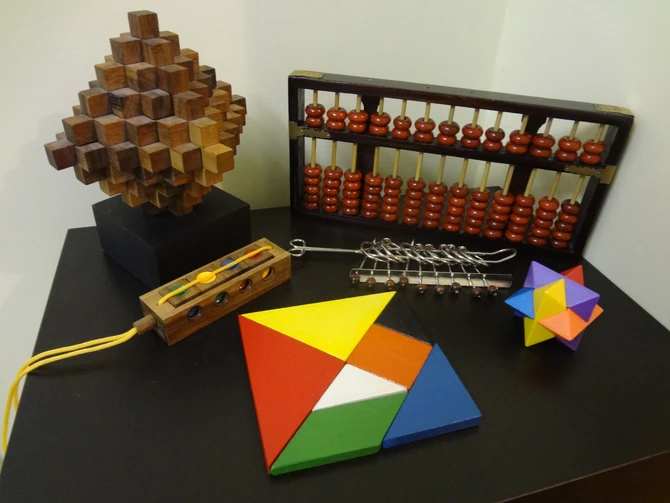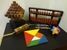## Welcome to the Math & Physics Problems Wiki!Welcome! This wiki is an archive of neat math, physics, chemistry, and computer programming problems that are great for high school and university students. My goal is to spread the art and culture of mathematical problem solving. Students who wish to navigate this wiki better (for practice problems) should check out the section Problem Sets of Steven Zheng below.

One of my areas of interest is the scientific history of ancient civilizations, especially the scientific development of the Orient. Check out History of STEM for insightful problems, interesting articles and interactive timelines on the history of STEM (Science, Technology, Engineering, Mathematics).

This wiki is also a portal for useful math and physics pages and free math/computation tools.

Want to learn math and physics better? Check out the pedagogy page.

## History of Mathematics

Europe
Egypt & Babylon
India & Islam
East Asia
Greek Mathematics
Egyptian Mathematics
Indian Mathematics
Chinese Mathematics
European Mathematics
Babylonian Mathematics
Islamic Mathematics
Japanese Mathematics## Problem Sets of Steven Zheng

Algebra
Geometry
Discrete Mathematics
Analytical Mathematics
Physics
Arithmetic and Conversions
Proportion
Number Theory
Differentiation
Mechanics
Linear Equations
Right Triangles
Sets and Logic
Integration
Electromagnetism and Optics
Measurement
Sequences and Series
Multivariable and Vector Calculus
Thermodynamics
Linear Algebra
Trigonometry
Counting and Probability
Complex Variables
Chemistry
Abstract Algebra
Vectors
Probability and Statistics
Differential Equations
Modern Physics

## Featured Articles

Eigenstates of an Infinite Square Well Read More...